×#### Thank you for registering.

One of our academic counsellors will contact you within 1 working day.

Click to Chat

1800-1023-196

+91-120-4616500

CART 0

• 0

MY CART (5)

Use Coupon: CART20 and get 20% off on all online Study Material

ITEM
DETAILS
MRP
DISCOUNT
FINAL PRICE
Total Price: Rs.

There are no items in this cart.
Continue Shopping• Complete JEE Main/Advanced Course and Test Series
• OFFERED PRICE: Rs. 15,900
• View Details

```Chapter 8: Quadratic Equations Exercise – 8.4

Question: 1

By using the method of completing the square, find the roots of quadratic equations.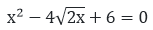Solution: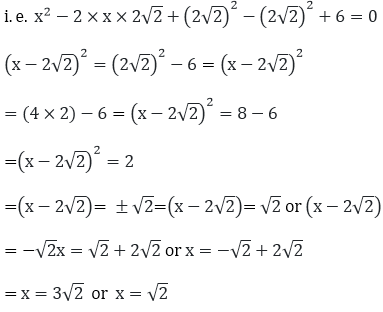So, the roots for the given equation are: x = 3√2or x = √2.

Question: 2

By using the method of completing the square, find the roots of quadratic equations.

2x2 − 7x + 3 = 0

Solution:

2x2 - 7x + 3 = 0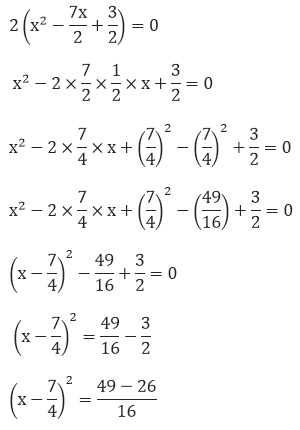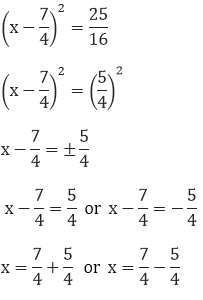x = 12/4 or x = 2/4

x = 3 or x = ½

Question: 3

By using the method of completing the square, find the roots of quadratic equations.

3x2 + 11x + 10 = 0

Solution:

3x2+ 11x + 10 = 0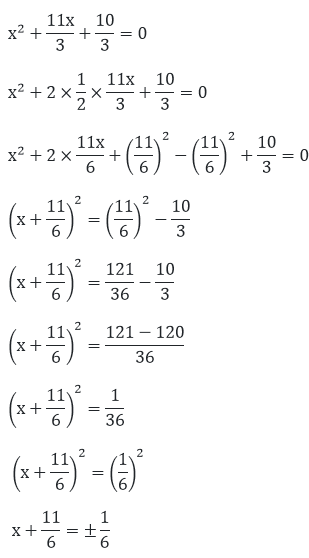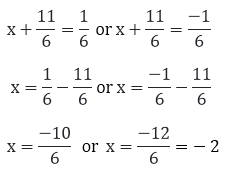x = (-5)/3 or x = - 2

Question: 4

By using the method of completing the square, find the roots of quadratic equations.

2x2 + x − 4 = 0

Solution:

2x2 + x − 4 = 0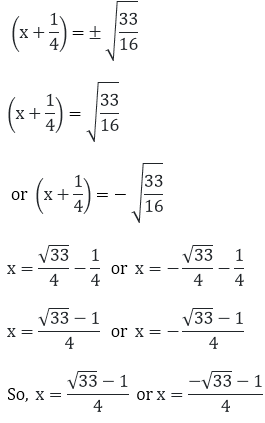Are the two roots of the given equation.

Question: 5

By using the method of completing the square, find the roots of quadratic equations.

2x2 + x + 4 = 0

Solution:

2x2 + x + 4 = 0

x2 + x2 + 2 = 0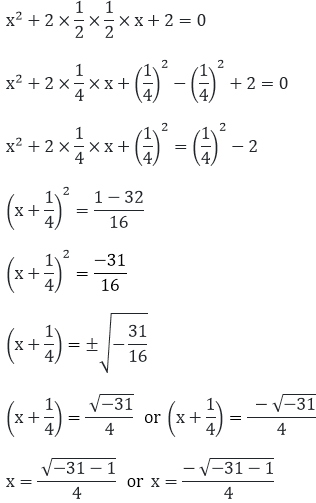Since, √(-31)  is not a real number, Therefore, the equation doesn’t have real roots.

Question: 6

By using the method of completing the square, find the roots of quadratic equations.Solution: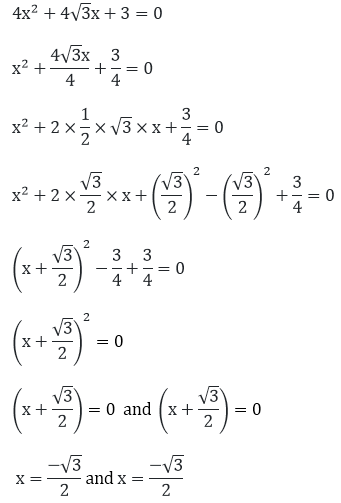Therefore, x = (- √3)/2 and x = (- √3)/2. Are the real roots of the given equation.

Question: 7

By using the method of completing the square, find the roots of quadratic equations.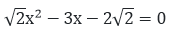Solution: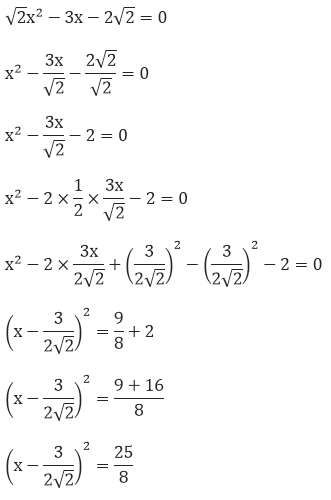Question: 8

By using the method of completing the square, find the roots of quadratic equations.Solution: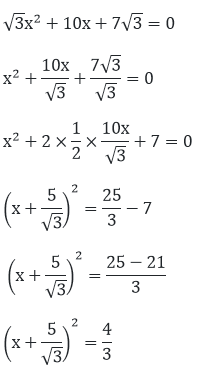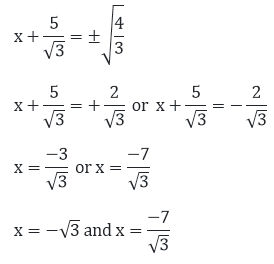Question: 9

By using the method of completing the square, find the roots of quadratic equations.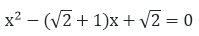Solution: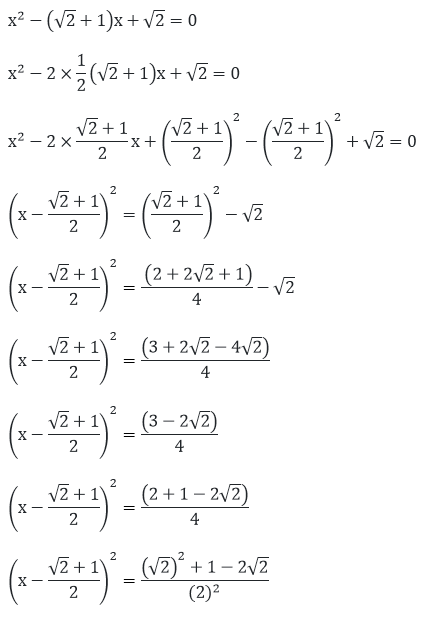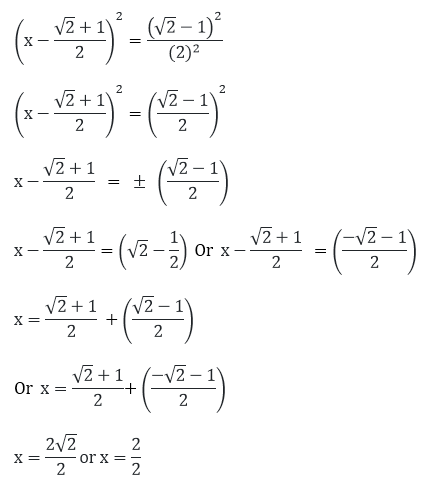x = √2  or  x = 1.

Question: 10

By using the method of completing the square, find the roots of quadratic equations.

x2 - 4ax + 4a2 - b2 = 0

Solution:

x2 - 4ax + 4a2 - b2 = 0

x2 - 2(2a).x + (2a)2 - b2 = 0

(x - 2a)2 = b2 x - 2a = ± b x - 2a = b or x - 2a

= - b x = 2a + b or  x = 2a - b

Therefore, x = 2a + b or  x = 2a - b are the two roots of the given equation.
```### Course Features

• 728 Video Lectures
• Revision Notes
• Previous Year Papers
• Mind Map
• Study Planner
• NCERT Solutions
• Discussion Forum
• Test paper with Video Solution